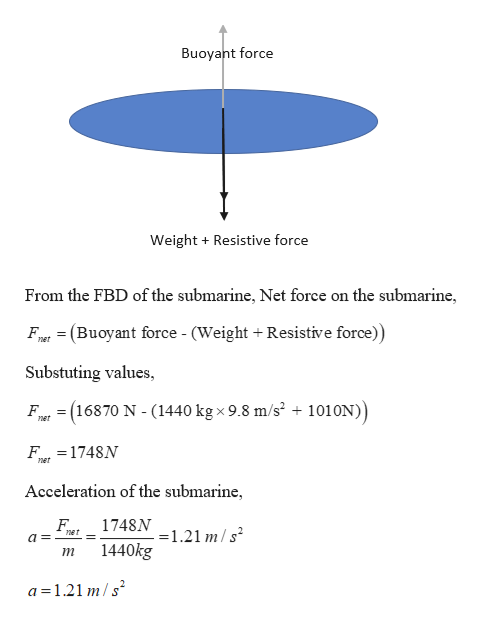A 1440-kg submarine rises straight up towards the surface. Seawater exerts both an upward buoyant force of 16870 N on the submarine, as well as a downward resistive force of 1010 N. What is the submarine's acceleration?

Question

A 1440-kg submarine rises straight up towards the surface. Seawater exerts both an upward buoyant force of 16870 N on the submarine, as well as a downward resistive force of 1010 N. What is the submarine's acceleration?

Step 1

Given:

Mass of the submarine = 1440 kg

Buoyant force in upward direction = 16870 N

Resistive force by the water = 1010 N

Step 2

Calculating the acceleratio...help_outlineImage TranscriptioncloseBuoyant force Weight Resistive force From the FBD of the submarine, Net force on the submarine, Ft (Buoyant force - (Weight + Resistive force)) net Substuting values = (16870 N - (1440 kgx 9.8 m/s2 + 1010N)) F ner F 1748N ner Acceleration of the submarine 1748N 121 m/s het 1440kg т a 1.21 m/s fullscreen

Want to see the full answer?

See Solution

Want to see this answer and more?

Our solutions are written by experts, many with advanced degrees, and available 24/7

See Solution
Tagged in

Fluid Mechanics